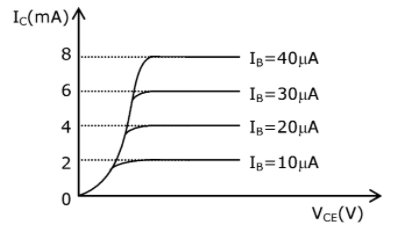# The typical output characteristics curve for a transistor working in the common emitter configuration is shown in the figureThe estimated current gain from the figure is …………..

For common emitter configuration

$$\begin{array}{l}\beta =\frac{\Delta I_{c}}{\Delta I_{B}}= \frac{(4-2)mA}{(20-10)\mu A}= \frac{2\times 10^{-3}}{10\times 10^{-6}}= 200\end{array}$$(2)(0)